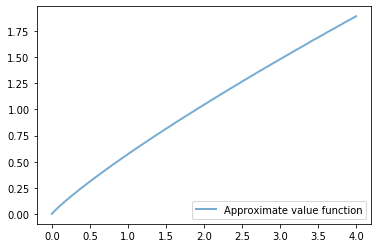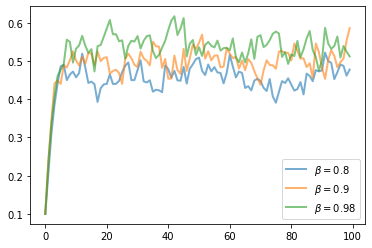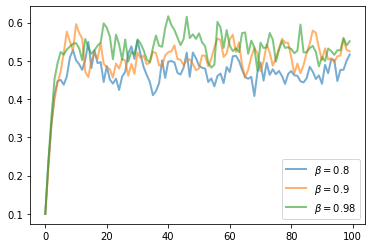• Lectures
• Code
• Notebooks
• Community

# Optimal Growth II: Accelerating the Code with Numba¶

## Contents¶

In addition to what’s in Anaconda, this lecture will need the following libraries:

In :
!pip install --upgrade quantecon


## Overview¶

In a previous lecture, we studied a stochastic optimal growth model with one representative agent.

We solved the model using dynamic programming.

In writing our code, we focused on clarity and flexibility.

These are good things but there’s often a trade-off between flexibility and speed.

The reason is that, when code is less flexible, we can exploit structure more easily.

(This is true about algorithms and mathematical problems more generally: more specific problems have more structure, which, with some thought, can be exploited for better results.)

So, in this lecture, we are going to accept less flexibility while gaining speed, using just-in-time compilation to accelerate our code.

In :
import numpy as np
import matplotlib.pyplot as plt
from interpolation import interp
from numba import jit, njit, jitclass, prange, float64, int32
from quantecon.optimize.scalar_maximization import brent_max

%matplotlib inline


We are using an interpolation function from interpolation.py because it helps us JIT-compile our code.

The function brent_max is also designed for embedding in JIT-compiled code.

These are alternatives to similar functions in SciPy (which, unfortunately, are not JIT-aware).

## The Model¶

The model is the same as discussed in this lecture.

We will use the same algorithm to solve it—the only difference is in the implementation itself.

We will use the CRRA utility specification

$$u(c) = \frac{c^{1 - γ} - 1} {1 - γ}$$

We continue to assume that

• $f(k) = k^{\alpha}$
• $\phi$ is the distribution of $\exp(\mu + s \zeta)$ when $\zeta$ is standard normal

## Computation¶

As before, we will store the primitives of the optimal growth model in a class.

But now we are going to use Numba’s @jitclass decorator to target our class for JIT compilation.

Because we are going to use Numba to compile our class, we need to specify the types of the data:

In :
opt_growth_data = [
('α', float64),          # Production parameter
('β', float64),          # Discount factor
('μ', float64),          # Shock location parameter
('γ', float64),          # Preference parameter
('s', float64),          # Shock scale parameter
('grid', float64[:]),    # Grid (array)
('shocks', float64[:])   # Shock draws (array)
]


Note the convention for specifying the types of each argument.

Now we’re ready to create our class, which will combine parameters and a method that realizes the right hand side of the Bellman equation (9).

You will notice that, unlike in the previous lecture, we hardwire the Cobb-Douglas production and CRRA utility specifications into the class.

Thus, we are losing flexibility, but we will gain substantial speed.

In :
@jitclass(opt_growth_data)
class OptimalGrowthModel:

def __init__(self,
α=0.4,
β=0.96,
μ=0,
s=0.1,
γ=1.5,
grid_max=4,
grid_size=120,
shock_size=250,
seed=1234):

self.α, self.β, self.γ, self.μ, self.s = α, β, γ, μ, s

# Set up grid
self.grid = np.linspace(1e-5, grid_max, grid_size)

# Store shocks (with a seed, so results are reproducible)
np.random.seed(seed)
self.shocks = np.exp(μ + s * np.random.randn(shock_size))

def f(self, k):
return k**self.α

def u(self, c):
return (c**(1 - self.γ) - 1) / (1 - self.γ)

def objective(self, c, y, v_array):
"""
Right hand side of the Bellman equation.
"""

u, f, β, shocks = self.u, self.f, self.β, self.shocks

v = lambda x: interp(self.grid, v_array, x)

return u(c) + β * np.mean(v(f(y - c) * shocks))


### The Bellman Operator¶

Here’s a jitted function that implements the Bellman operator

In :
@jit(nopython=True)
def T(og, v):
"""
The Bellman operator.

* og is an instance of OptimalGrowthModel
* v is an array representing a guess of the value function
"""
v_new = np.empty_like(v)

for i in range(len(og.grid)):
y = og.grid[i]

# Maximize RHS of Bellman equation at state y
v_max = brent_max(og.objective, 1e-10, y, args=(y, v))
v_new[i] = v_max

return v_new


Here’s another function, very similar to the last, that computes a $v$-greedy policy:

In :
@jit(nopython=True)
def get_greedy(og, v):
"""
Compute a v-greedy policy.

* og is an instance of OptimalGrowthModel
* v is an array representing a guess of the value function
"""
v_greedy = np.empty_like(v)

for i in range(len(og.grid)):
y = og.grid[i]

# Find maximizer of RHS of Bellman equation at state y
c_star = brent_max(og.objective, 1e-10, y, args=(y, v))
v_greedy[i] = c_star

return v_greedy


The last two functions could be merged, as they were in our previous implementation, but we resisted doing so to increase efficiency.

Here’s a function that iterates from a starting guess of the value function until the difference between successive iterates is below a particular tolerance level.

In :
def solve_model(og,
tol=1e-4,
max_iter=1000,
verbose=True,
print_skip=25):

# Set up loop
v = np.log(og.grid)  # Initial condition
i = 0
error = tol + 1

while i < max_iter and error > tol:
v_new = T(og, v)
error = np.max(np.abs(v - v_new))
i += 1
if verbose and i % print_skip == 0:
print(f"Error at iteration {i} is {error}.")
v = v_new

if i == max_iter:
print("Failed to converge!")

if verbose and i < max_iter:
print(f"\nConverged in {i} iterations.")

return v_new


Let’s compute the approximate solution at the default parameters.

First we create an instance:

In :
og = OptimalGrowthModel()


Now we call solve_model, using the %%time magic to check how long it takes.

In :
%%time
v_solution = solve_model(og)

Error at iteration 25 is 0.9732383838286296.
Error at iteration 50 is 0.2005788181409116.
Error at iteration 75 is 0.07224367966546197.
Error at iteration 100 is 0.026036371917143697.
Error at iteration 125 is 0.009383422955124843.
Error at iteration 150 is 0.0033817548269325926.
Error at iteration 175 is 0.0012187733382234.
Error at iteration 200 is 0.00043924190907773664.
Error at iteration 225 is 0.00015830134196193058.

Converged in 237 iterations.
CPU times: user 6.95 s, sys: 19.6 ms, total: 6.97 s
Wall time: 6.97 s


You will notice that this is much faster than our original implementation.

Let’s plot the resulting policy:

In :
v_greedy = get_greedy(og, v_solution)

fig, ax = plt.subplots()

ax.plot(og.grid, v_greedy, lw=2,
alpha=0.6, label='Approximate value function')

ax.legend(loc='lower right')
plt.show()Everything seems in order, so our code acceleration has been successful!

## Exercises¶

### Exercise 1¶

Once an optimal consumption policy $\sigma$ is given, income follows

$$y_{t+1} = f(y_t - \sigma(y_t)) \xi_{t+1}$$

The next figure shows a simulation of 100 elements of this sequence for three different discount factors (and hence three different policies)In each sequence, the initial condition is $y_0 = 0.1$.

The discount factors are discount_factors = (0.8, 0.9, 0.98).

We have also dialed down the shocks a bit with s = 0.05.

Otherwise, the parameters and primitives are the same as the log-linear model discussed earlier in the lecture.

Notice that more patient agents typically have higher wealth.

Replicate the figure modulo randomness.

## Solutions¶

### Exercise 1¶

Here’s one solution

In :
def simulate_og(σ_func, og, y0=0.1, ts_length=100):
'''
Compute a time series given consumption policy σ.
'''
y = np.empty(ts_length)
ξ = np.random.randn(ts_length-1)
y = y0
for t in range(ts_length-1):
y[t+1] = (y[t] - σ_func(y[t]))**og.α * np.exp(og.μ + og.s * ξ[t])
return y

In :
fig, ax = plt.subplots()

for β in (0.8, 0.9, 0.98):

og = OptimalGrowthModel(β=β, s=0.05)

v_solution = solve_model(og)
v_greedy = get_greedy(og, v_solution)

# Define an optimal policy function
σ_func = lambda x: interp(og.grid, v_greedy, x)
y = simulate_og(σ_func, og)
ax.plot(y, lw=2, alpha=0.6, label=rf'$\beta = {β}$')

ax.legend(loc='lower right')
plt.show()

Error at iteration 25 is 0.012245923836417205.

Converged in 44 iterations.
Error at iteration 25 is 0.20961181523261985.
Error at iteration 50 is 0.008387575216147525.
Error at iteration 75 is 0.0006017314226482995.

Converged in 93 iterations.
Error at iteration 25 is 1.636332251620388.
Error at iteration 50 is 0.5549102065497209.
Error at iteration 75 is 0.3346444091976082.
Error at iteration 100 is 0.20194598162470356.
Error at iteration 125 is 0.12186727717256929.
Error at iteration 150 is 0.07354260348984099.
Error at iteration 175 is 0.04438036734114803.
Error at iteration 200 is 0.026781986385216783.
Error at iteration 225 is 0.016161984176847.
Error at iteration 250 is 0.009753187414219155.
Error at iteration 275 is 0.005885704607351272.
Error at iteration 300 is 0.003551815140596659.
Error at iteration 325 is 0.0021433951642393367.
Error at iteration 350 is 0.00129346338508185.
Error at iteration 375 is 0.0007805595314920311.
Error at iteration 400 is 0.0004710401467207248.
Error at iteration 425 is 0.0002842561149236644.
Error at iteration 450 is 0.00017153853966078714.
Error at iteration 475 is 0.00010351745852688055.

Converged in 477 iterations.• Share page